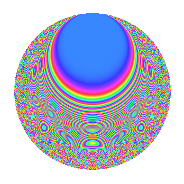# Properties

 Label 8045.2.a.cLevel 8045 Weight 2 Character orbit 8045.a Self dual Yes Analytic conductor 64.240 Analytic rank 1 Dimension 127 CM No

# Related objects

## Newspace parameters

 Level: $$N$$ = $$8045 = 5 \cdot 1609$$ Weight: $$k$$ = $$2$$ Character orbit: $$[\chi]$$ = 8045.a (trivial)

## Newform invariants

 Self dual: Yes Analytic conductor: $$64.2396484261$$ Analytic rank: $$1$$ Dimension: $$127$$ Fricke sign: $$1$$ Sato-Tate group: $\mathrm{SU}(2)$

## $q$-expansion

The dimension is sufficiently large that we do not compute an algebraic $$q$$-expansion, but we have computed the trace expansion.

 $$\operatorname{Tr}(f)(q) =$$ $$127q$$ $$\mathstrut -\mathstrut 20q^{2}$$ $$\mathstrut -\mathstrut 31q^{3}$$ $$\mathstrut +\mathstrut 116q^{4}$$ $$\mathstrut +\mathstrut 127q^{5}$$ $$\mathstrut -\mathstrut 17q^{6}$$ $$\mathstrut -\mathstrut 63q^{7}$$ $$\mathstrut -\mathstrut 57q^{8}$$ $$\mathstrut +\mathstrut 122q^{9}$$ $$\mathstrut +\mathstrut O(q^{10})$$ $$\operatorname{Tr}(f)(q) =$$ $$127q$$ $$\mathstrut -\mathstrut 20q^{2}$$ $$\mathstrut -\mathstrut 31q^{3}$$ $$\mathstrut +\mathstrut 116q^{4}$$ $$\mathstrut +\mathstrut 127q^{5}$$ $$\mathstrut -\mathstrut 17q^{6}$$ $$\mathstrut -\mathstrut 63q^{7}$$ $$\mathstrut -\mathstrut 57q^{8}$$ $$\mathstrut +\mathstrut 122q^{9}$$ $$\mathstrut -\mathstrut 20q^{10}$$ $$\mathstrut -\mathstrut 32q^{11}$$ $$\mathstrut -\mathstrut 65q^{12}$$ $$\mathstrut -\mathstrut 49q^{13}$$ $$\mathstrut -\mathstrut 4q^{14}$$ $$\mathstrut -\mathstrut 31q^{15}$$ $$\mathstrut +\mathstrut 98q^{16}$$ $$\mathstrut -\mathstrut 53q^{17}$$ $$\mathstrut -\mathstrut 60q^{18}$$ $$\mathstrut -\mathstrut 126q^{19}$$ $$\mathstrut +\mathstrut 116q^{20}$$ $$\mathstrut -\mathstrut 24q^{21}$$ $$\mathstrut -\mathstrut 46q^{22}$$ $$\mathstrut -\mathstrut 121q^{23}$$ $$\mathstrut -\mathstrut 51q^{24}$$ $$\mathstrut +\mathstrut 127q^{25}$$ $$\mathstrut -\mathstrut 9q^{26}$$ $$\mathstrut -\mathstrut 112q^{27}$$ $$\mathstrut -\mathstrut 123q^{28}$$ $$\mathstrut -\mathstrut 6q^{29}$$ $$\mathstrut -\mathstrut 17q^{30}$$ $$\mathstrut -\mathstrut 54q^{31}$$ $$\mathstrut -\mathstrut 119q^{32}$$ $$\mathstrut -\mathstrut 57q^{33}$$ $$\mathstrut -\mathstrut 53q^{34}$$ $$\mathstrut -\mathstrut 63q^{35}$$ $$\mathstrut +\mathstrut 133q^{36}$$ $$\mathstrut -\mathstrut 61q^{37}$$ $$\mathstrut -\mathstrut 27q^{38}$$ $$\mathstrut -\mathstrut 33q^{39}$$ $$\mathstrut -\mathstrut 57q^{40}$$ $$\mathstrut -\mathstrut 8q^{41}$$ $$\mathstrut -\mathstrut 46q^{42}$$ $$\mathstrut -\mathstrut 208q^{43}$$ $$\mathstrut -\mathstrut 54q^{44}$$ $$\mathstrut +\mathstrut 122q^{45}$$ $$\mathstrut -\mathstrut 34q^{46}$$ $$\mathstrut -\mathstrut 116q^{47}$$ $$\mathstrut -\mathstrut 106q^{48}$$ $$\mathstrut +\mathstrut 110q^{49}$$ $$\mathstrut -\mathstrut 20q^{50}$$ $$\mathstrut -\mathstrut 54q^{51}$$ $$\mathstrut -\mathstrut 142q^{52}$$ $$\mathstrut -\mathstrut 60q^{53}$$ $$\mathstrut -\mathstrut 62q^{54}$$ $$\mathstrut -\mathstrut 32q^{55}$$ $$\mathstrut +\mathstrut 33q^{56}$$ $$\mathstrut -\mathstrut 56q^{57}$$ $$\mathstrut -\mathstrut 87q^{58}$$ $$\mathstrut -\mathstrut 53q^{59}$$ $$\mathstrut -\mathstrut 65q^{60}$$ $$\mathstrut -\mathstrut 76q^{61}$$ $$\mathstrut -\mathstrut 84q^{62}$$ $$\mathstrut -\mathstrut 215q^{63}$$ $$\mathstrut +\mathstrut 67q^{64}$$ $$\mathstrut -\mathstrut 49q^{65}$$ $$\mathstrut +\mathstrut 9q^{66}$$ $$\mathstrut -\mathstrut 145q^{67}$$ $$\mathstrut -\mathstrut 133q^{68}$$ $$\mathstrut +\mathstrut q^{69}$$ $$\mathstrut -\mathstrut 4q^{70}$$ $$\mathstrut -\mathstrut 4q^{71}$$ $$\mathstrut -\mathstrut 167q^{72}$$ $$\mathstrut -\mathstrut 155q^{73}$$ $$\mathstrut -\mathstrut 14q^{74}$$ $$\mathstrut -\mathstrut 31q^{75}$$ $$\mathstrut -\mathstrut 199q^{76}$$ $$\mathstrut -\mathstrut 97q^{77}$$ $$\mathstrut -\mathstrut 24q^{78}$$ $$\mathstrut -\mathstrut 73q^{79}$$ $$\mathstrut +\mathstrut 98q^{80}$$ $$\mathstrut +\mathstrut 127q^{81}$$ $$\mathstrut -\mathstrut 69q^{82}$$ $$\mathstrut -\mathstrut 225q^{83}$$ $$\mathstrut -\mathstrut 59q^{84}$$ $$\mathstrut -\mathstrut 53q^{85}$$ $$\mathstrut +\mathstrut 30q^{86}$$ $$\mathstrut -\mathstrut 179q^{87}$$ $$\mathstrut -\mathstrut 119q^{88}$$ $$\mathstrut -\mathstrut 25q^{89}$$ $$\mathstrut -\mathstrut 60q^{90}$$ $$\mathstrut -\mathstrut 160q^{91}$$ $$\mathstrut -\mathstrut 188q^{92}$$ $$\mathstrut -\mathstrut 44q^{93}$$ $$\mathstrut -\mathstrut 32q^{94}$$ $$\mathstrut -\mathstrut 126q^{95}$$ $$\mathstrut -\mathstrut 43q^{96}$$ $$\mathstrut -\mathstrut 72q^{97}$$ $$\mathstrut -\mathstrut 111q^{98}$$ $$\mathstrut -\mathstrut 141q^{99}$$ $$\mathstrut +\mathstrut O(q^{100})$$

## Embeddings

For each embedding $$\iota_m$$ of the coefficient field, the values $$\iota_m(a_n)$$ are shown below.

For more information on an embedded modular form you can click on its label.

Label $$a_{2}$$ $$a_{3}$$ $$a_{4}$$ $$a_{5}$$ $$a_{6}$$ $$a_{7}$$ $$a_{8}$$ $$a_{9}$$ $$a_{10}$$
1.1 −2.82452 −1.42855 5.97791 1.00000 4.03498 −1.55315 −11.2357 −0.959233 −2.82452
1.2 −2.74468 −3.15686 5.53326 1.00000 8.66457 −4.01431 −9.69765 6.96577 −2.74468
1.3 −2.72254 3.22119 5.41223 1.00000 −8.76982 −3.61577 −9.28994 7.37607 −2.72254
1.4 −2.71791 −0.717872 5.38703 1.00000 1.95111 −2.87590 −9.20565 −2.48466 −2.71791
1.5 −2.69637 1.21455 5.27040 1.00000 −3.27487 2.45597 −8.81819 −1.52487 −2.69637
1.6 −2.69512 −2.02504 5.26369 1.00000 5.45774 1.98108 −8.79604 1.10080 −2.69512
1.7 −2.68498 2.75441 5.20913 1.00000 −7.39554 −2.94460 −8.61647 4.58677 −2.68498
1.8 −2.61813 0.911081 4.85463 1.00000 −2.38533 −2.60374 −7.47379 −2.16993 −2.61813
1.9 −2.53777 0.243430 4.44030 1.00000 −0.617771 4.53173 −6.19292 −2.94074 −2.53777
1.10 −2.52291 −2.56612 4.36506 1.00000 6.47407 −1.96305 −5.96681 3.58496 −2.52291
1.11 −2.51055 −0.644369 4.30288 1.00000 1.61772 −0.493808 −5.78150 −2.58479 −2.51055
1.12 −2.49863 2.41476 4.24316 1.00000 −6.03361 1.44699 −5.60483 2.83108 −2.49863
1.13 −2.47054 −3.37029 4.10358 1.00000 8.32645 4.71800 −5.19700 8.35885 −2.47054
1.14 −2.44032 0.296251 3.95518 1.00000 −0.722948 −5.11077 −4.77127 −2.91224 −2.44032
1.15 −2.41609 −2.71250 3.83748 1.00000 6.55363 −4.44734 −4.43951 4.35764 −2.41609
1.16 −2.35235 −3.18470 3.53353 1.00000 7.49152 −1.35488 −3.60740 7.14233 −2.35235
1.17 −2.31881 0.00298266 3.37687 1.00000 −0.00691620 −0.956165 −3.19269 −2.99999 −2.31881
1.18 −2.26786 −1.17957 3.14318 1.00000 2.67510 2.15242 −2.59257 −1.60862 −2.26786
1.19 −2.26133 1.69211 3.11360 1.00000 −3.82641 −1.09235 −2.51822 −0.136771 −2.26133
1.20 −2.24197 1.72389 3.02644 1.00000 −3.86491 −1.20930 −2.30126 −0.0282099 −2.24197
See next 80 embeddings (of 127 total)
 $$n$$: e.g. 2-40 or 990-1000 Embeddings: e.g. 1-3 or 1.127 Significant digits: Format: Complex embeddings Normalized embeddings Satake parameters Satake angles

## Inner twists

This newform does not have CM; other inner twists have not been computed.

## Atkin-Lehner signs

$$p$$ Sign
$$5$$ $$-1$$
$$1609$$ $$-1$$

## Hecke kernels

This newform can be constructed as the kernel of the linear operator $$T_{2}^{127} + \cdots$$ acting on $$S_{2}^{\mathrm{new}}(\Gamma_0(8045))$$.